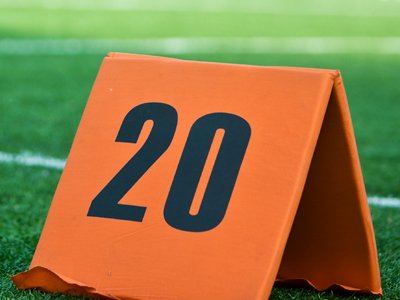2 × 10 = 20.

# 2 Times Table

This Math quiz is called '2 Times Table' and it has been written by teachers to help you if you are studying the subject at elementary school. Playing educational quizzes is an enjoyable way to learn if you are in the 3rd, 4th or 5th grade - aged 8 to 11.

It costs only \$12.50 per month to play this quiz and over 3,500 others that help you with your school work. You can subscribe on the page at Join Us

The 'Two Times' table is important because the time taken for something to double, the doubling time, is an important piece of information in a lot of practical situations, e.g. inflation, population growth and compound interest.

See if you can double your scores in this quiz.

1.
2 × 3 = ?
8
4
6
5
2.
2 × 7 = ?
10
12
14
16
3.
2 × 8 = ?
16
12
18
14
4.
2 × 9 = ?
18
19
14
16
5.
2 × 6 = ?
16
14
10
12
6.
2 × 11 = ?
14
18
22
26
7.
2 × 4 = ?
6
8
4
12
8.
2 × 2 = ?
1
6
8
4
9.
2 × 12 = ?
22
24
26
20
10.
2 × 5 = ?
12
8
12
10
Author:  Frank Evans